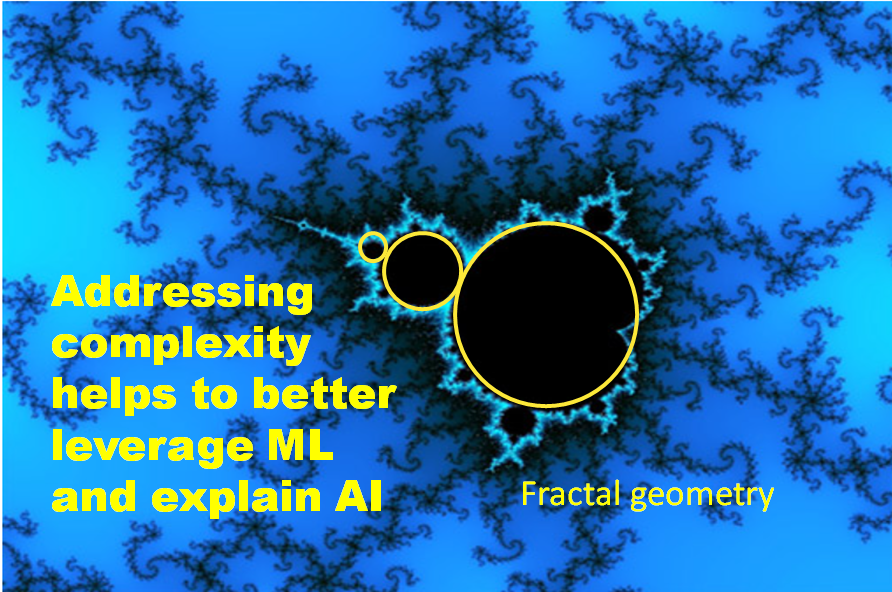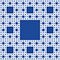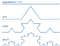# Addressing complexity helps to better leverage ML and explain AIMandelbrot fractal set. Benoit Mandelbrot is a pioneer of fractal geometry — Hunting the Hidden Dimension — PBS

Both ML and AI involve feature extraction procedures, either explicitly or implicitly, such as shape or texture features extraction. However, such features often exhibit complex and fractal behavior that can be a challenge to capture or extract using Euclidean geometry.

Addressing such complexity using feature engineering based on fractal geometry can help tremendously to better leverage ML and explain AI. This was based on an early work that I have conducted with colleagues using Matlab and Neural Network with fractals to capture features of gene expression morphogenesis. Fractals with ML helped us to capture subtle variation in gene expression when compared with Euclidean geometry.

Fractals is helping even more vastly in today’s information technology with its use in mobile phone. Fractal-based models are helping in the description and classification of scale-related phenomena in life sciences, from molecular to higher ecosystem levels of organization.Sierpinski Carpet fractal used in mobile phone antenna as the most efficient way of receiving signals when compared to traditional Euclidian antenna.

The Sierpiński carpet is a fractal two-diension plane named after Sierpiński (1916). The carpet is as generalization of the Cantor set to two dimensions and it is generated by subdividing a 2D shape into smaller copies of itself, removing one or more copies recursively. Cantor set is used to detect error in computer networks and transmission via communication networks along with lossless data compression algorithms.

Fractal feature extraction procedures

Fractal features can be captured as dimensions (D) to detect whether data exhibit patterns and surface roughness in engineering and industry. The fractal dimension such as box counting (Db) is measured by counting the numbers of boxes occupied by the black pixels of black and white images. The box size started first with 2 large squares on one side, and then 2n squares, with n varying depending on the box size.

Commonly used to illustrate a fractal dimension is the Koch curve, which is a typical geometric fractal that is generated by starting with an equilateral triangle, then continue adding other equilateral triangles pointing outwards in the middle of each side of subsequent triangle, recursively.Koch Curve fractal has a D value of = 1.26185, a fractal dimension between a line and a plane — 1 (line) < D = 1.26185 < 2 (plane)

There are several other procedures to capture shape and texture in addition to box counting where Db is then estimated by plotting the number of boxes N(d) against the box-side length d. Some methods used are similar to the areography technique in which boxes are fixed ‘sub-regions” defined by geographical coordinates instead of grids of changing sizes.

Since the box counting values can change with re-orientation and the relatively low resolution of digitized images it could produce an underestimation of counts for smaller boxes, the variogram approach can be used instead to capture fractal dimensions (Dv).

The variogram fractal dimension Dv has a link to the Hurst exponent, which is commonly referred to by H. The standard deviation SD2(h) of the difference between points (Z(i) and Z(0)) of the object, which follows a normal distribution, is proportional to H (h2H). The Hurst exponent is a measure used in time series and also commonly used to capture trends in financial data. Similar to AUC when H=0.5 indicates a random series and when AUC >0.5 as indication of presence of a pattern in data in the case of H>0.5 it is an indication of presence of a trend supporting times series.

Modelling complex gene expression — mRNA

A number of networked genes are commonly involved with gene expression. The expression of some genes may increase or decrease the expression of others, forming a complex network of interactions. There are a number of such complex networks of genes involving genotype-by-environment interaction as well as epistatic interaction between genes regulating variation for gene expression. The interaction and functioning among these networks of genes could be predicted if the said networks were sufficiently understood and appropriately quantified. The gene network models are based on the expression of gene i as a level of mRNA, at time with value X, among N genes, represented by a connection matrix M, and at a subsequent time with a new value, X’ = MX (complexity). In addition to the capture of complex traits based on quantitative modelling of features such as fractals, quantitative models for predicting functioning of gene networks can help to predict phenotypes that may emerge from gene expression dynamics.

mRNA stands for messenger ribonucleic acid, which is a single-stranded molecule of RNA that corresponds to the genetic sequence of a gene. mRNA has been used to develop vaccine to protect against COVID 19.

References

Some of the work conducted using fractals and neural networks to capture gene expression can be found in Complexity and Fractals in Nature at https://www.worldscientific.com/worldscibooks/10.1142/6032

OperAI develops IoTs with Math and AI Embedded Solutions to speed up and streamline operational processes at the edges of the cloud.

## More from OperAI - Operational Artificial Intelligence

OperAI develops IoTs with Math and AI Embedded Solutions to speed up and streamline operational processes at the edges of the cloud.